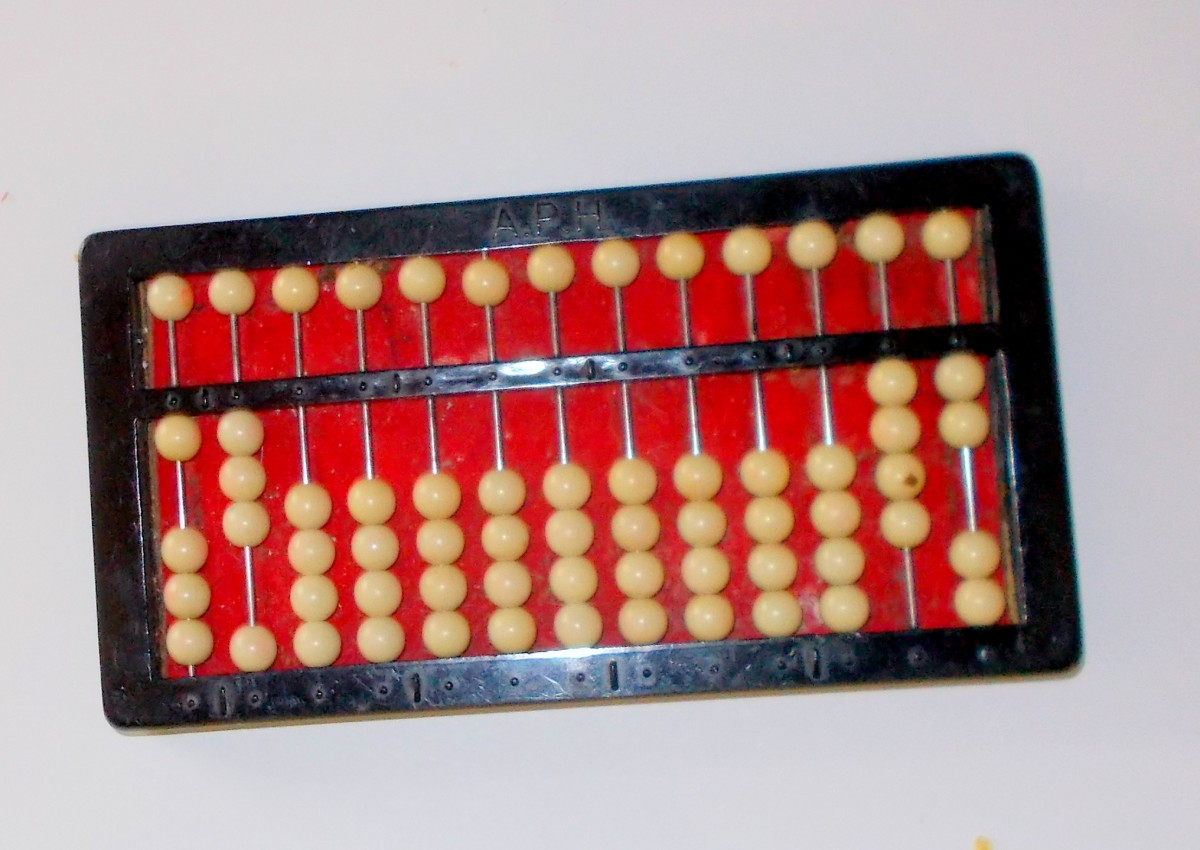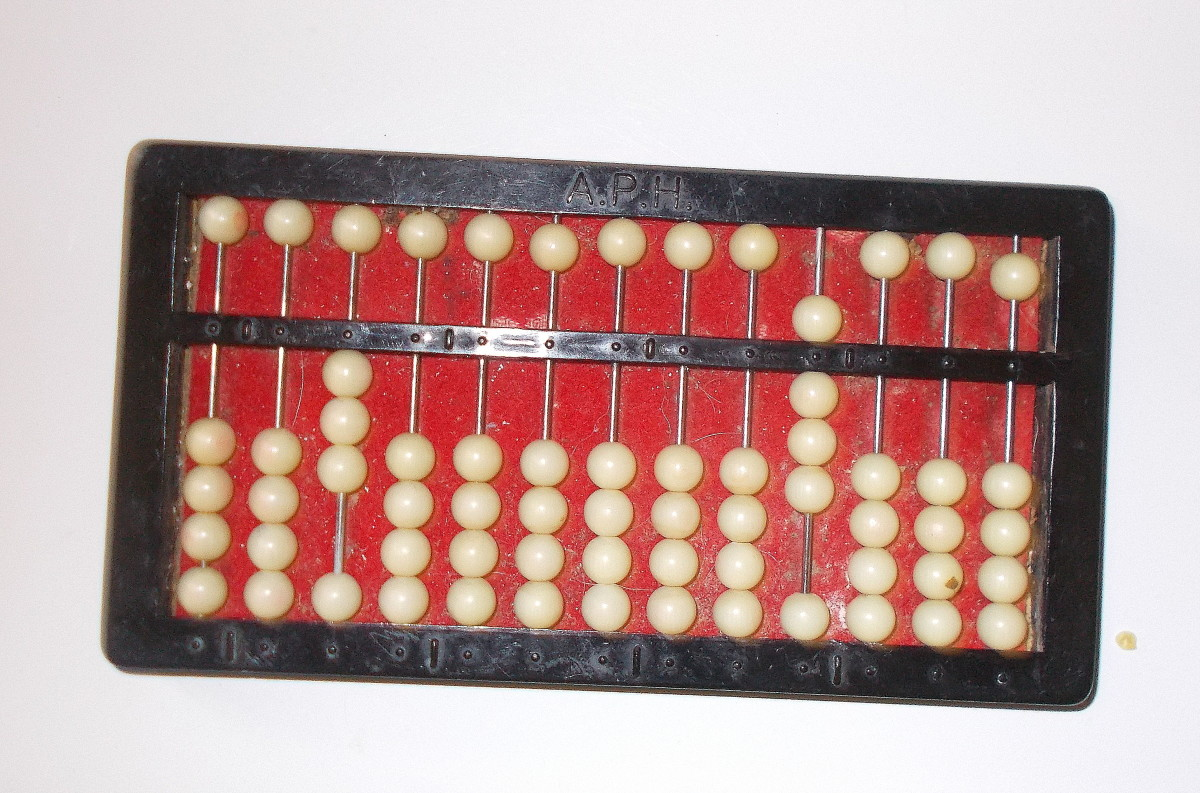# Easy Steps on How to Multiply Fractions on the Abacus

Tim Truzy is a rehabilitation counselor, educator, and former dispatcher from North Carolina.

## The Abacus and Fractions

The abacus is versatile enough to carry out any number of mathematical processes. Whether working with addition, subtraction, multiplication or division, a person with the proper knowledge can effectively find answers using the counting tool. This includes working with whole numbers or fractions. Whole numbers are positive integers without pieces, or fractional parts. The infinite set of whole numbers can be expressed in this manner: {0, 1, 2, 3, 4, … {. Normally, these numbers can easily be set on the abacus.

By contrast, fractions are composed of numbers representing part of the whole. Much like whole numbers, fractions can be shown on the abacus as well. Common fractions, also known as simple fractions, take the form of a/b. The number zero cannot be the denominator, represented by the letter B in this example. However, applying concepts relevant to operating the abacus can render the same results as from other techniques.

Although there are different abaci, I used the Cranmer abacus for this article and others. Years of study and instructing students have allowed me to develop techniques for working with various mathematical processes on the counting device. Nevertheless, regular practice is required to master the abacus. Below is an approach for performing multiplication of fractions on the abacus. To begin, bring your abacus to rest, like in the first photo in this article. Soon we will be multiplying fractions using the fascinating counting device.

## Knowledge to Review

1. Using the relevant terms while correctly conducting mathematical operations with the abacus is definitely important. These terms include: keeping balance, pay back, at rest, and one for the abacus. Setting whole numbers on the counting tool should not present a challenge for the user of the abacus if he/she is ready to work with fractions. By this point, a person should have successfully completed addition and subtraction problems using the abacus before attempting to multiply fractions.
2. In addition, having the knowledge to properly carry out multiplication and division problems should be firmly established. The user of the abacus should know his/her multiplication tables through the number nine. Working knowledge of division should be present, including comprehension of crucial terms such as quotient. Before going forward with fractions, a person should have solved multiplication and division problems with whole numbers using the abacus as well.
3. Finally, a basic understanding of what a fraction represents needs to be a part of the abacus user’s knowledge. Understanding and applying the concept of mentally “splitting” the abacus at the halfway point of the device should be a comfortable cognitive task. Now, let’s set a fraction and get ready to multiply using the abacus.

## Setting Our First Fraction

• Fractions are composed of three parts: the numerator, the division symbol, and the denominator. The abacus in the picture shows the fraction: one-half.
• We set the numerator, 1, on the farthest column to the left.
• We set the denominator, 2, in the ones’ column on the right. This is the first column going from right to left on the counting tool.
• This is how fractions are placed on the abacus.
• Notice: In setting fractions on the abacus, we must mentally split the counting tool much as is done with division and multiplication. Now, bring the abacus to rest.This abacus is showing the fraction one-half multiplied by the fraction ¾.Tim Truzy

## Multiplying Two Fractions

1. First, set the equation: 1/2 x 3/4. Your abacus should resemble the photo above.
2. Recognize that the numbers on the left represent two numerators: 1 and 3. (This is not the number: 13. It’s important to always remember what mathematical process you are performing at any time using any method of solving equations)
3. Also, recognize the numbers on the left represent two denominators: 2 and 4. )This is not the number: 42.)
4. Now, multiply the numerators: 1 x 3. Your product will be 3.
5. Next, move two columns to the right. In essence, you will skip a row of beads, and place your first product: 3. This is “giving one for the abacus.)
6. Now, place the 3 there.
7. Multiply the denominators: 4 x 2. Your answer will be 8.
8. Finally, move two columns from the 4, and place the denominator: 8.
9. Here, you “gave one for the abacus” as well.
10. Now, clear ½ and ¾.This is the fraction which is the answer for the equation ½ x ¾. The abacus is showing 3/8.Tim Truzy

## Explanation for Giving One for the Abacus when Multiplying Fractions

Generally in western culture we are not taught to think of (0)+1, (0)+2, etc. when we count. Basically, the concept of "giving one for the abacus" signifies the number is less than ten. This concept becomes easier when you have an abacus in front of you, then that (0) becomes an empty column of beads you can touch.

In the example above, we moved over two columns before placing both products. This process is done because the products are less than ten. Therefore, the products are counted as (0) plus 3 for the numerators, and (0) plus 8 for the denominators.

In other words, the zero represents the empty column. With products greater than ten, giving one for the abacus becomes unnecessary. Now, let’s prepare to perform another equation multiplying fractions.

Scroll to Continue

## Let's Solve Another Equation with Fractions on the Abacus

1. Set 1/7 on your abacus.
2. Now, set 3/5. You have set the equation: 1/7 x 3/5. It should look like the photo.
3. Next, multiply the numerators: 1 x 3. Your answer is 3.
4. Count one row of beads for the abacus as 0, and place the 3 on the fourth row from the far left.
5. Now, multiply the denominators: 5 x 7. The product is 35.
6. Working from the right side of the counting tool, immediately next to the two denominators, you count: 3 tens for one row, and 5 ones for the next row.
7. Here, you would place 35 on the fourth and third columns of beads.
8. Now, clear the equation: 3/5 x 1/7.
9. Your answer is 3/35. It should look like the photo below.
11. Congratulations. You have successfully multiplied fractions using the abacus.

## Poll

Tim Truzy (author) from U.S.A. on September 28, 2018:

Thanks, Flourish,

But as gifted as you are, I know you would catch the hang of it. Don't feel bad about that concept - my wife, who is also proficient on the abacus - still grits her teeth at the concept of "giving one for the abacus."

It's mainly because we are not taught to think of (0)+1, (0)+2, etc. when we count. Basically, the concept signifies the number is less than 10.

It's easier when you have an abacus in front of you, then that (0) becomes an empty column of beads you can touch.

I always appreciate you as a writer and a kind human being,

Sincerely,

Tim

FlourishAnyway from USA on September 27, 2018:

I bet you’re a great teacher. I had a bit of a problem with the giving one to the abacus but was able to follow your examples.

Tim Truzy (author) from U.S.A. on September 27, 2018:

This article explores how the abacus can be applied to solving multiplication problems with fractions. It can be confusing, especially if time and practice is not given to developing the learning senses with the number machine. In order to become more proficient with the counting tool, mentally dividing the abacus is a must when working with fractions. However, I've helped many students accomplish this task. You can, too.